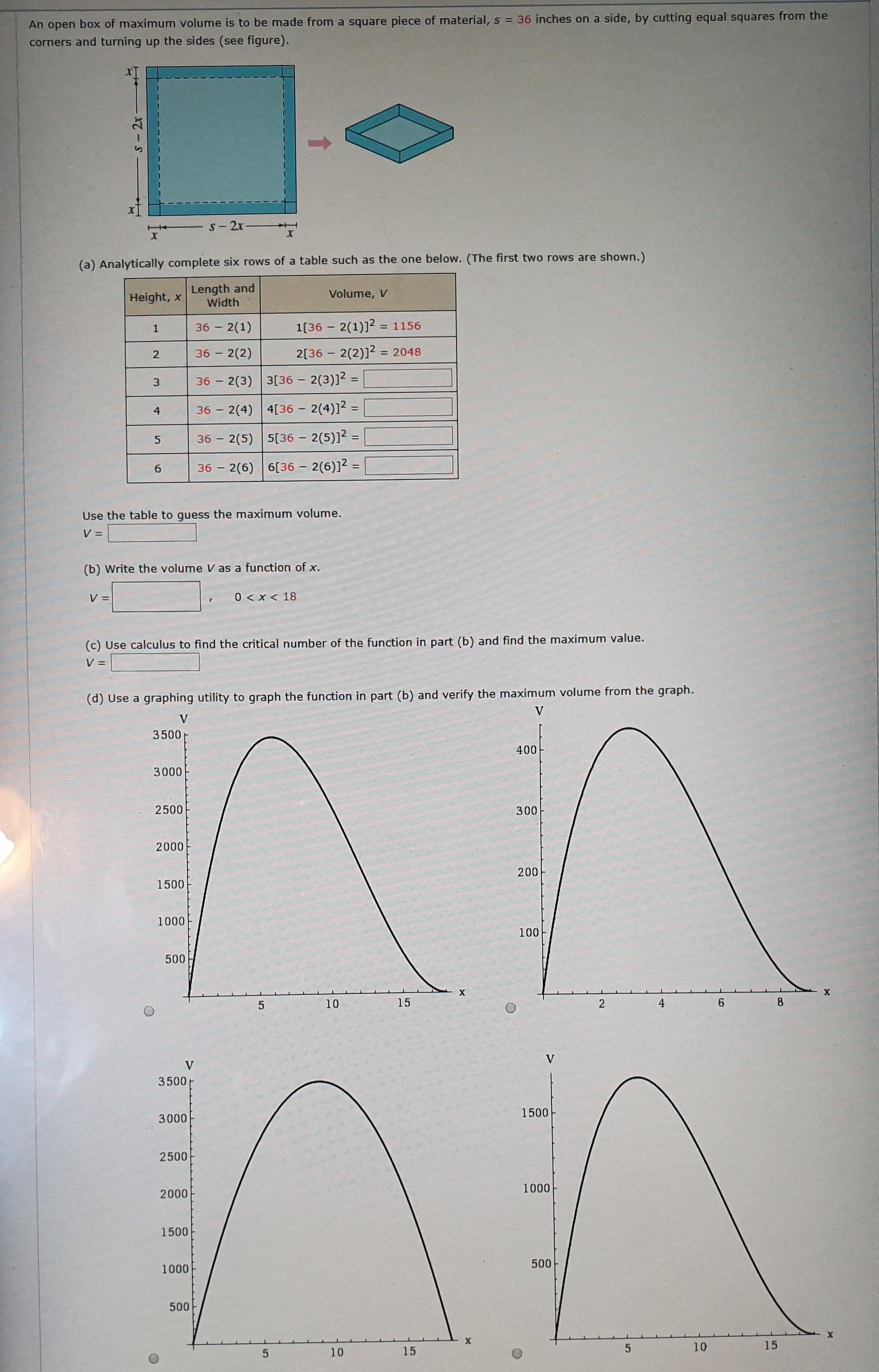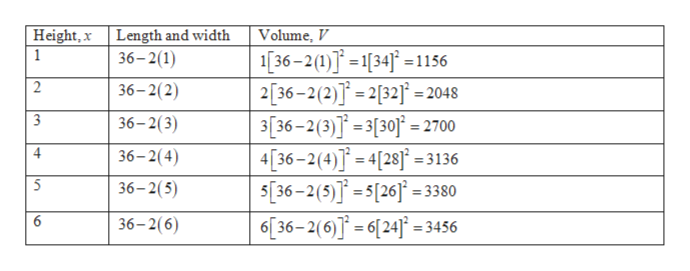# a side, by cutting equal squares from theAn open box of maximum volume is to be made from a square piece of material, s = 36 inches oncorners and turning up the sides (see figure).S-2r-TXshown.)(a) Analytically complete six rows of a table such as the one below. (The first two rows areLength andWidthVolume, VHeight, x1[36 2(1)]22(1)11563612[36 2(2)]22(2)= 2048363[36 2(3)]236 2(3)34[36 2(4)]2 =36 2(4)2(5) 5[36 2(5)]2 =3636 2(6) 6[36 2(6)12 -Use the table to guess the maximum volume.V=(b) Write the volume V as a function of x.0 x< 18V=c) Use calculus to find the critical number of the function in part (b) and find the maximum value.V =(d) Use a graphing utility to graph the function in part (b) and verify the maximum volume from the graph.VV350040030002500300200020015001000100500XX154102VV35001500300025001000200015005001000500X15101510st

Question
29 views

How can I get the result?

Which is the result?help_outlineImage Transcriptionclosea side, by cutting equal squares from the An open box of maximum volume is to be made from a square piece of material, s = 36 inches on corners and turning up the sides (see figure). S-2r- T X shown.) (a) Analytically complete six rows of a table such as the one below. (The first two rows are Length and Width Volume, V Height, x 1[36 2(1)]2 2(1) 1156 36 1 2[36 2(2)]2 2(2) = 2048 36 3[36 2(3)]2 36 2(3) 3 4[36 2(4)]2 = 36 2(4) 2(5) 5[36 2(5)]2 = 36 36 2(6) 6[36 2(6)12 - Use the table to guess the maximum volume. V= (b) Write the volume V as a function of x. 0 x< 18 V= c) Use calculus to find the critical number of the function in part (b) and find the maximum value. V = (d) Use a graphing utility to graph the function in part (b) and verify the maximum volume from the graph. V V 3500 400 3000 2500 300 2000 200 1500 1000 100 500 X X 15 4 10 2 V V 3500 1500 3000 2500 1000 2000 1500 500 1000 500 X 15 10 15 10 st fullscreen
check_circle

Step 1

(a)

Complete the table below analytically:help_outlineImage TranscriptioncloseHeight, xLength and width 36-2(1 Volume, V 36-2(1) 341156 236-2(2)]=2[32= 2048 3[36-2(3)]3[30= 2700 4 36-2(4)283136 S[36-2(5)][263380 6[36-2(6)]24 3456 1 2 36-2(2) 3 36-2(3) 4 36-2(4) 5 36-2(5) 6 36-2(6) re fullscreen
Step 2

From the table, the maximum volume is 3456 cubic units.

Step 3

(b)

As length and width of box is (s-2x ) = (36-2x ) each and height is x...

### Want to see the full answer?

See Solution

#### Want to see this answer and more?

Solutions are written by subject experts who are available 24/7. Questions are typically answered within 1 hour.*

See Solution
*Response times may vary by subject and question.
Tagged in

### Other